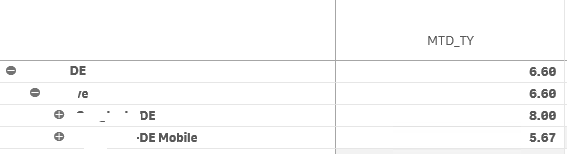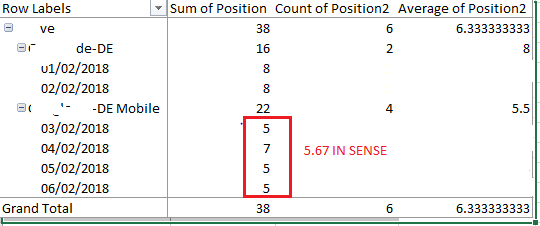# New to Qlik Sense

If you’re new to Qlik Sense, start with this Discussion Board and get up-to-speed quickly.

Announcements
Welcome to Qlik Community! Check out our new navigation! FIND OUT MORE
cancel
Showing results for
Did you mean:Contributor II

## Pivot table understanding indent rows aggregation with average - help

Hello guys,

I am using the Pivot Table object to aggregate some data in the latest version of Qlik Sense enterprise version.

Data is being aggregated by average.

The problem is that I cannot understand how qlik is showing the indent Rows

As per below pic:

I have a total avg of my sample set as 6.6.

This sample has 2 subsamples (DE and DE Mobile) which have 8 and 5.67 as average respectively.the pivot above is derived from a small sample of 6 rows.

Full sample extract from excel below.

De Mobile has 4 rows and adds up to 22 as per below I would expect to have an average of 5.5 instead of 5.67.Furthermore, the total average is set in sens at 6.6 but would expect to see 6.3.

The 6.6 returned seems to exclude the last row of DE Mobile so that there is a sum of 33 and a count of 5 giving an avg of 6.6, but why should be that. Am I missing something? In the straight table, I do not see duplicates or anything else I should consider here.

Thank you in advance,

Francesco

1 Solution

Accepted SolutionsMVP

I think averages can get tricky... look here Average – Which average?

You might be able to do this

Sum({\$< _MTD_TY = {1}, Pos={'>=1<=10'}>}Pos)/Count(DISTINCT {\$< _MTD_TY = {1}, Pos={'>=1<=10'}>} DateField)

4 RepliesMVP

What expression are you using in your chart for Sum of Position? What all dimensions do you have?Contributor II
Author

Hi Sunny,

Thanks for your question...the Sum of Position has no expression. What I am trying to show is that in excel I presented all the rows that should have an average of 5.5 while sense is presenting as 5.67.

In qlik sense the 5.67 is given by a set analysis expression: Avg({\$< _MTD_TY = {1}, Pos={'>=1<=10'}>}Pos)

MTD TY should pick all the 4 rows in the excel as well as the filter Pos={'>=1<=10'} which only takes POS from 1 to 10 (all the rows are in this range).

Thanks,

FrancescoMVP

I think averages can get tricky... look here Average – Which average?

You might be able to do this

Sum({\$< _MTD_TY = {1}, Pos={'>=1<=10'}>}Pos)/Count(DISTINCT {\$< _MTD_TY = {1}, Pos={'>=1<=10'}>} DateField)Contributor II
Author

Thank you Sunny for your reply, I eventually found, thanks to your hint, where the QSense number in the pivot was coming from....the pivot takes into account some rows which should not be there ...I will not use avg in the pivot then but perhaps work with an expression like you have proposed. Marking the correct answer thanks.Tags
Community Browser